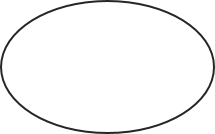# Ellipse Area Calculator

If you forgot how to find the area of an ellipse, you’re in the right place – this online ellipse area calculator is the answer to your problems. Play with the calculation tool, read on and refresh your memory to find out what is the area of an ellipse and to learn the formula behind the calculation.

## What is an ellipse?

An ellipse is a curve in a plane where the sum of the distances from any point on the curve to each of its two focal points is constant.## Ellipse area formula

The area of an ellipse can be obtained with the ellipse area formula below:

`area = a * b * π`

where a and b are the semi-major and semi-minor axes of the ellipse.

## How to find the area of an ellipse?

You can use the ellipse area calculator to find the area of an ellipse:

1. Enter the length of the semi-major axes (a).
2. Enter the length of the semi-minor axes (b).
3. Our ellipse calculation tool performs all the calculations for you. The area is displayed.

#### This calculator is created with

the best online spreadsheet editor with excellent formula and editing capability.

For more calculators for finance, mathematics health, unit converters and more check out our calculators collection.